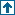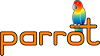parrotcode: Untitled Contents | Compilers

# FUNCTIONSThis file contains emit functions.

XXX Take the print_* function as a template to create the PBC-generating functions.

`void print_key(key *k)`

Print the key `k`. The total key is enclosed in square brackets, and different key elements are separated by semicolons. Example:

` ["hi";42]`
has two elements: `"hi"` and `42`.

`void print_target(target *const t)`

Print the target `t`; if `t` has a key, that key is printed as well. Examples:

` S1, P1`
`void print_constant(constant *c)`

Print the value of constant `c`.

`void print_expr(lexer_state *const lexer, expression *const expr)`

Print the expression `expr`.

`void print_expressions(expression *const expr)`

Print the list of expressions pointed to by `expr`, if `expr` is not NULL. Expressions are separated by commas.

`void print_subs(struct lexer_state *const lexer)`

`void emit_pbc(lexer_state *const lexer)`

Generate Parrot Byte Code from the abstract syntax tree. This is the top-level function; it initializes the data structures (code segment), and then starts emitting byte code (by means of helper functions).